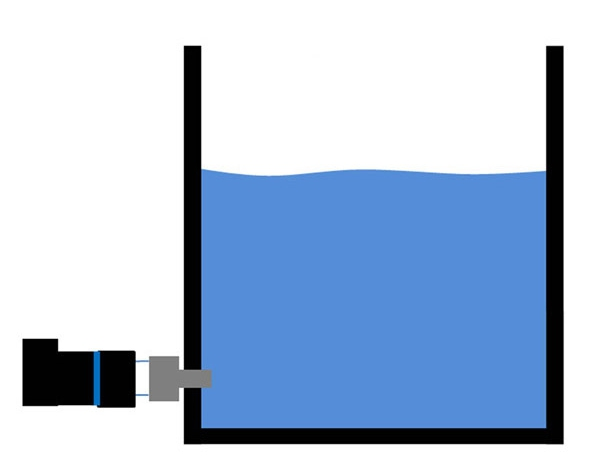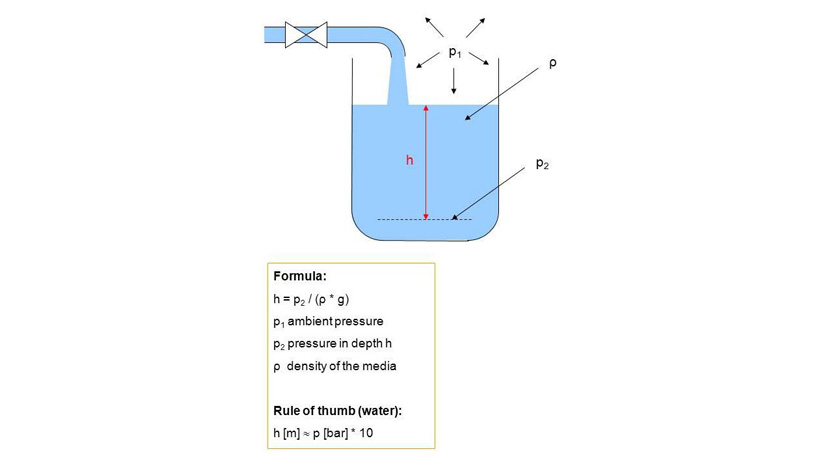PT PERTA MAHAKA INDONESIA "Manufacturer & Supplier of Instrument, Valve & Control System"
News & EventPT Perta Mahaka Indonesia
Jl. Raya Ciherang No. 166, Sukatani, Tapos, Depok - Jawa Barat 16454
(021) 874 5456
Customer Service Perta0811 147 5456

## How does hydrostatic level measurement work?

27/09/2018When considering a new level sensor for level measurement applications, it is often hard to find information on how the different types of level sensors, like the hydrostatic level sensors respectively hydrostatic pressure sensors, are working. Recently, I explained level measurement by using hydrostatic pressure measurement

“What does one mean by hydrostatic pressure and hydrostatic level measurement?” But how do you measure the level of water and other liquids using pressure sensors? For use in open geometries such as vented vessels, open reservoirs and tanks, but also wells and open bodies of water, instrumentation manufacturers such as WIKA offer submersible pressure transmitters, also known as level probes, specifically developed for this purpose. Submersible pressure transmitters are completely immersed in the liquid that is to be measured.

In closed geometries, such as enclosed tanks and vessels, generally, flush pressure sensors or pressure transmitters are used, which can be screwed into the vessel from the outside. Pressure sensors are therefore particularly suitable in applications of free-standing tanks and vessels. A submersible pressure transmitter (e.g. WIKA model LH-20) or a flush pressure sensor (e.g. WIKA model S-11) measures the height-dependent weight-force of the liquid column, also known as hydrostatic pressure. This pressure measurement is directly proportional to the liquid level respectively liquid column at the measuring point of the pressure sensor. It is therefore necessary, for the measurement of level, that the pressure sensor is placed as close as possible to the level of the vessel’s bottom.

The measured hydrostatic pressure then directly corresponds to the height of the liquid column from the ground-level measurement point to the surface-level of the medium. So, the filling height is calculated from the distance of the medium surface to the measuring point by the pressure measurement. The weight force of the liquid column, thus the hydrostatic pressure, however, is not only directly proportional to the filling height, but also varies with the specific gravity of the medium and the force of gravity. In the next article I will explain in detail the calculation of the liquid level, especially with reference to the influence of the specific gravity of the medium.## Calculation of the filling height using hydrostatic pressure

Under the influence of gravity, the hydrostatic pressure rises with the increasing height of the liquid column, thus with the filling height of the vessel.

Thus the level is calculated from the formula:

h = p / (ρ * g)

• p = hydrostatic pressure [bar gauge]
• ρ = density of the liquid [kg/m³]
• g = gravitational force or gravitational acceleration [m/s²]
• h = height of the liquid column [m]

Rule of thumb – Water:                h = 1 bar relative / (1000 kg/m³ * ~ 10 m/s²) = 10 m

For the medium water, one can thus adopt the rule of thumb that a pressure of 1 bar corresponds to a filling height of 10 m.

This rule of thumb can be used for selection and specification of a suitable submersible pressure sensor or pressure sensor. As a control, however, a more precise calculation should be performed, which includes the influence of temperature on the density, as well as the location-dependent force of gravity in the level calculation.

Since the density of a medium can significantly deviate from the density of water, this rule of thumb only applies to liquids with densities close to water. Thus, for example, with the same filling height of diesel and water, the hydrostatic pressure of diesel is much lower than that of water.

Example – Diesel fuel: h = 0.82 bar gauge / (820 kg/m³ * ~ 10 m/s²) = 10 m

The density difference has, in this example, led to a measuring error of around 22 %.

Since with hydrostatic level measurement in open basins and vessels, there is constant ventilation, that is to say, a pressure equalisation takes place between the gas above the liquid and the ambient air, the pressure of the overlying gas must not be included in the calculation of the level. By using submersible pressure sensors, such as the WIKA model LH-20, in a gauge pressure variant, the fluctuating ambient pressure is fully compensated.Level measurement in closed or pressurised, thus non-vented vessels requires an additional pressure measurement of the enclosed gas by a second pressure sensor. In a closed vessel, the pressure measurement is made, primarily, using standard industrial pressure sensors which are mounted at the side of the vessel or tank. Now, how does one calculate, from the hydrostatic pressure, the filling height of a closed, non-vented or pressurised tank or vessel?

The level in a closed vessel is calculated by the following formula: h = (p2 – p1)/ (ρ * g) p2 = hydrostatic pressure [bar] at depth h p1= pressure of the enclosed gas in the vessel [bar] ρ = density of the liquid [kg/m³] g = gravitational force or gravitational acceleration [m/s²] h = height of the liquid column [m] A typical application is  measuring the level of a volatile medium such as gasoline in a sealed, non-vented vessel, where an overpressure forms above the liquid, which can’t be compensated by the atmospheric pressure due to the lack of venting.

This pressure must be measured by a second pressure sensor, since the gas enclosed above the liquid results in a higher hydrostatic pressure measurement, even though no real change in level has occurred.

## Example of a false level calculation:

(Please use our pressure unit converter for a calculation by Psi, Pa and more) p2 (hydrostatic measurement at the bottom of the tank) = 2 bar p1 (gas pressure measurement) = 1.2 bar p1-typ (typical gas pressure) = 1.3 bar ρ = 750 kg/m³ g = 9.81 m/s² When compensating the hydrostatic measurement with the gas pressure, the liquid level can be measured very accurately using the hydrostatic pressure.

With gas pressure measurement:            h = (2 bar – 1.2 bar) / (750 kg/m³ * 9.81 m/s²) = 10.9 m

However, if you decide not to use an additional pressure measurement for the enclosed gas, if you work with an estimated value, e.g. based on the typical prevailing gas pressure, even small pressure fluctuations in the process lead to major miscalculations of the level.

Without gas pressure measurement:     h = (2 bar – 1.3 bar) / (750 kg/m³ * 9.81 m/s²) = 9.5 m

So, even a small change of 100 mbar in the gas pressure may lead to a large measuring error of the liquid level, like 13 % in this example. Specifically a lower measurement could result in a process-critical error such as overflow of the medium from the tank, or the contamination of following vessels in the process chain.

It is therefore imperative to compensate for the additional gas pressure within the level calculation in enclosed, unventilated or pressurised vessels.

(Enrico Bossart-WIKA)

INFORMATION

CONTACT

PT Perta Mahaka Indonesia
Jl. Raya Ciherang No. 166, Sukatani, Tapos, Depok - Jawa Barat 16454
(021) 874 5456
Customer Service Perta0811 147 5456